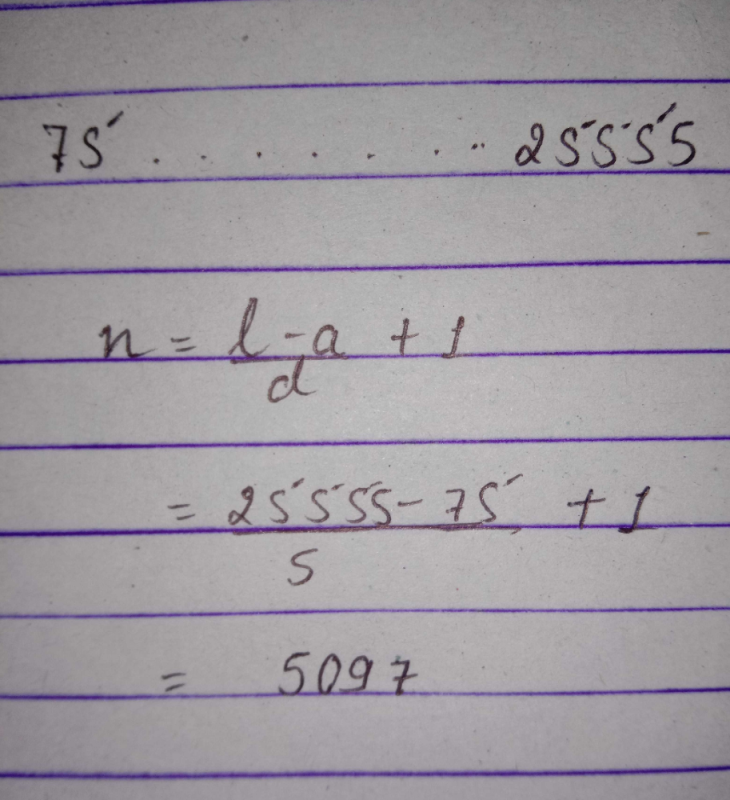Courses

# The number of numbers between 74 and 25556 divisible by 5 isa)5090b)5097c)5095d)none of theseCorrect answer is option 'B'. Can you explain this answer? Related Test: Test: Arithmetic And Geometric Progressions - 1

## CA Foundation QuestionKhushi Sahu Oct 12, 2020Mayank Sharma Nov 23, 2018
Let a be the first term and d be the common difference.
First term a = 75.
Common difference = 5
Last term an = 25555.
We know that number of terms of an AP an = a + (n - 1) * d
25555 = 75 + (n - 1) * 5
25555 = 75 + 5n - 5
25555 = 70 + 5n
25485 = 5n
n = 5097.
Therefore there are 5097 numbers between 74 and 25556 divisible by 5.Ann Maria Sunil Sep 22, 2020
Answer =5097
A=75,last term=25555,d=5
We know that
25555=75+(n-1)5
(n-1)5=25555-75
n-1=25480÷5
n-1=5096
n=5097

This discussion on The number of numbers between 74 and 25556 divisible by 5 isa)5090b)5097c)5095d)none of theseCorrect answer is option 'B'. Can you explain this answer? is done on EduRev Study Group by CA Foundation Students. The Questions and Answers of The number of numbers between 74 and 25556 divisible by 5 isa)5090b)5097c)5095d)none of theseCorrect answer is option 'B'. Can you explain this answer? are solved by group of students and teacher of CA Foundation, which is also the largest student community of CA Foundation. If the answer is not available please wait for a while and a community member will probably answer this soon. You can study other questions, MCQs, videos and tests for CA Foundation on EduRev and even discuss your questions like The number of numbers between 74 and 25556 divisible by 5 isa)5090b)5097c)5095d)none of theseCorrect answer is option 'B'. Can you explain this answer? over here on EduRev! Apart from being the largest CA Foundation community, EduRev has the largest solved Question bank for CA Foundation.